Print
Rate 0 stars
Common Core
• Lesson size:
Message preview:
Someone you know has shared lesson with you:

To play this lesson, click on the link below:

Hope you have a good experience with this site and recommend to your friends too.

Login to rate activities and track progress.
Login to rate activities and track progress.

Michelle has \$3.52 in lunch money and Steven has \$2.78. How much money do they have altogether?

To solve problems like this, we need to learn to add or subtract decimals.

Adding and subtracting decimals is simple - it's just like adding and subtracting whole numbers!

First, line up your numbers so that the decimal points are in the same place: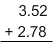Ignore the decimal points for a bit - just pretend they're not there and add as you would with whole numbers.

Then, put in the decimal point exactly below the other decimal points, so that all of your decimal points line up vertically.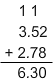So, the total money with Michelle and Steven is \$3.52 + \$2.78 = \$6.30.

Let's take a look at some more examples.

## Example 1

Subtract: 6.7 - 3.5

First, line up your numbers so that the decimal points are in the same place:Ignore the decimal points for a bit - just pretend they're not there and subtract as you would with whole numbers.

Then, put in the decimal point exactly below the other decimal points, so that all of your decimal points line up verticallySo, 6.7 - 3.5 = 3.2.

Note:

You can use this approach even if your decimals have different numbers of digits - just remember to line up the decimal points, and fill in any blanks with zeros.

## Example 2

Nina ran 0.761 mile on Tuesday and 0.4 mile on Wednesday. How many miles did she run over the two days?

First, line up your numbers so that the decimal points are in the same place: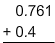Next, fill in the blanks with zeros.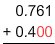Then, add the two numbers just like you would add two whole numbers. Finally, put the decimal point.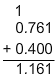So, Nina ran 1.161 miles over the two days.

Summary

Steps to add or subtract decimals:

• First, line up the decimals so that the decimal points are in the same place.
• If the decimals have different numbers of digits, remember to line up the decimal points, and fill in the blanks with zeros so that the decimals have the same number of places.
• Add or subtract, as you would with whole numbers.
• In the answer, put in the decimal point exactly below the other decimal points, so that all of the decimal points line up vertically.

## More Decimals Lessons

Become premium member to get unlimited access.